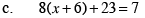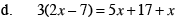### Home > CAAC > Chapter 7 > Lesson 7.1.4 > Problem7-39

7-39.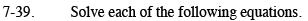Note: Some steps may be done differently.
Be sure to check you answers by substituting them into the original equations.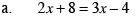Subtract 2x from both sides.

8 = x − 4

12 = x

Verify that 2(12) + 8 = 3(12) − 4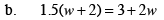Use the Distributive Property.

1.5w + 3 = 3 + 2w

Subtract 1.5x from both sides.

Subtract 3 from both sides.

Divide both sides by 0.5.

w = 0